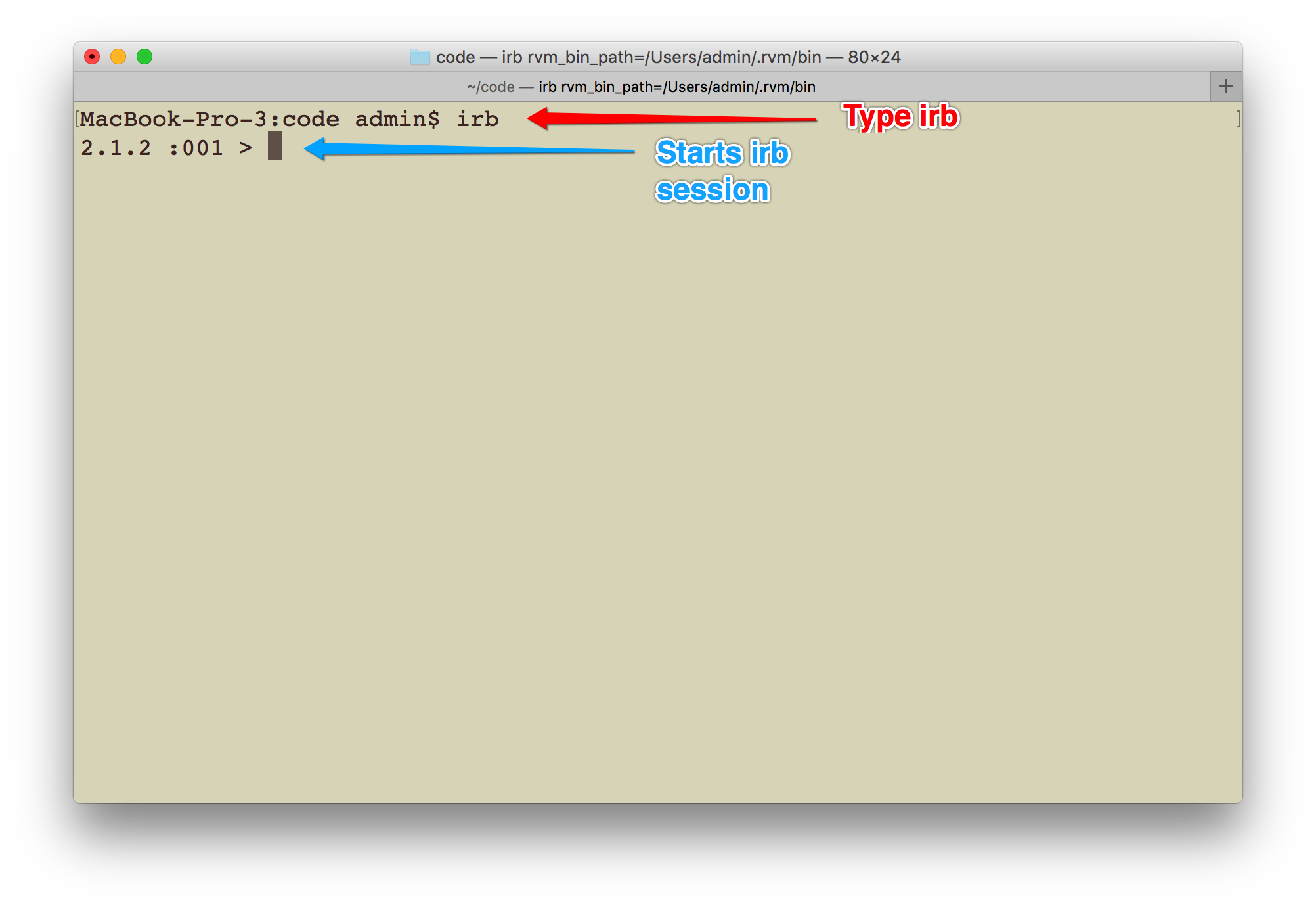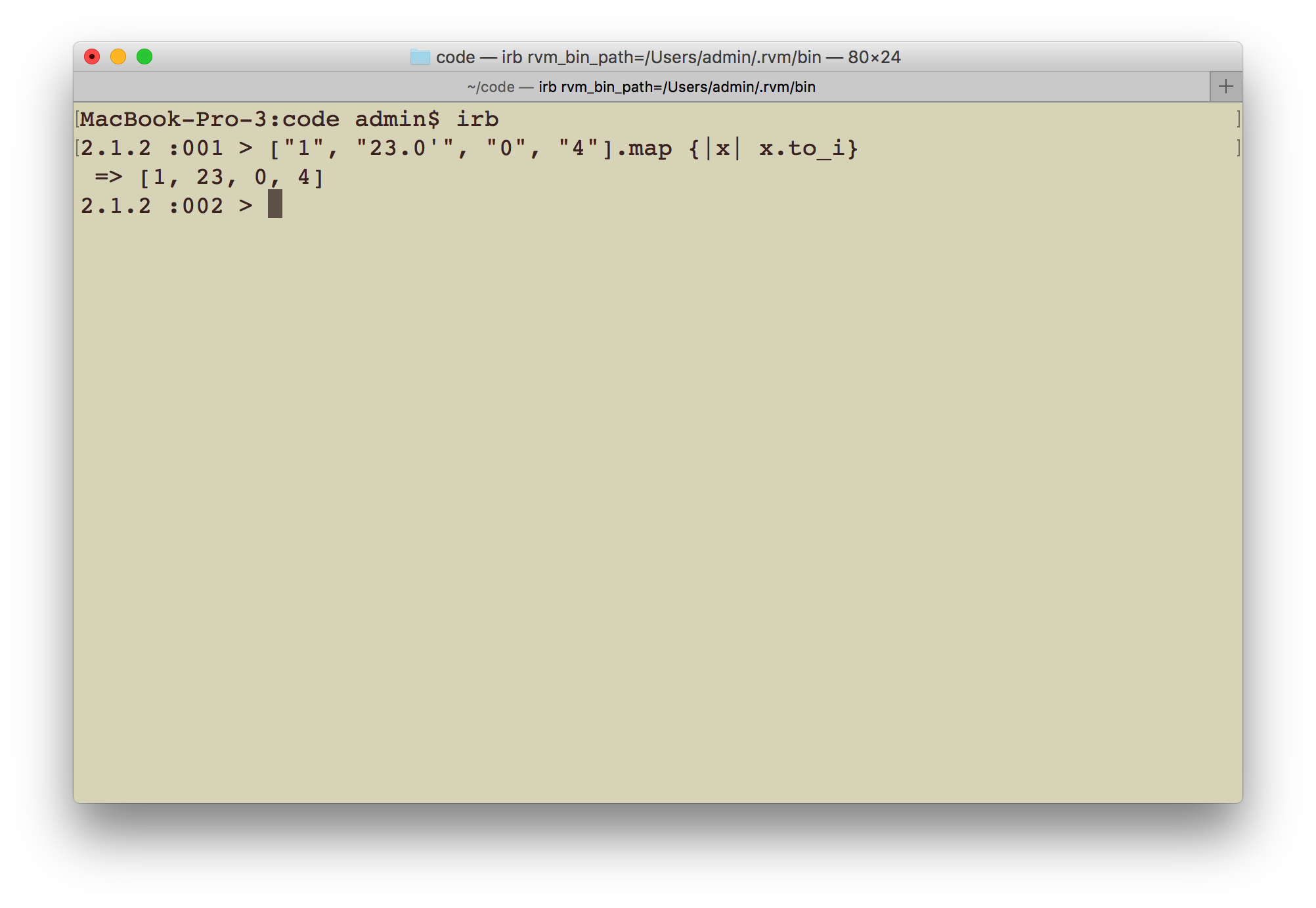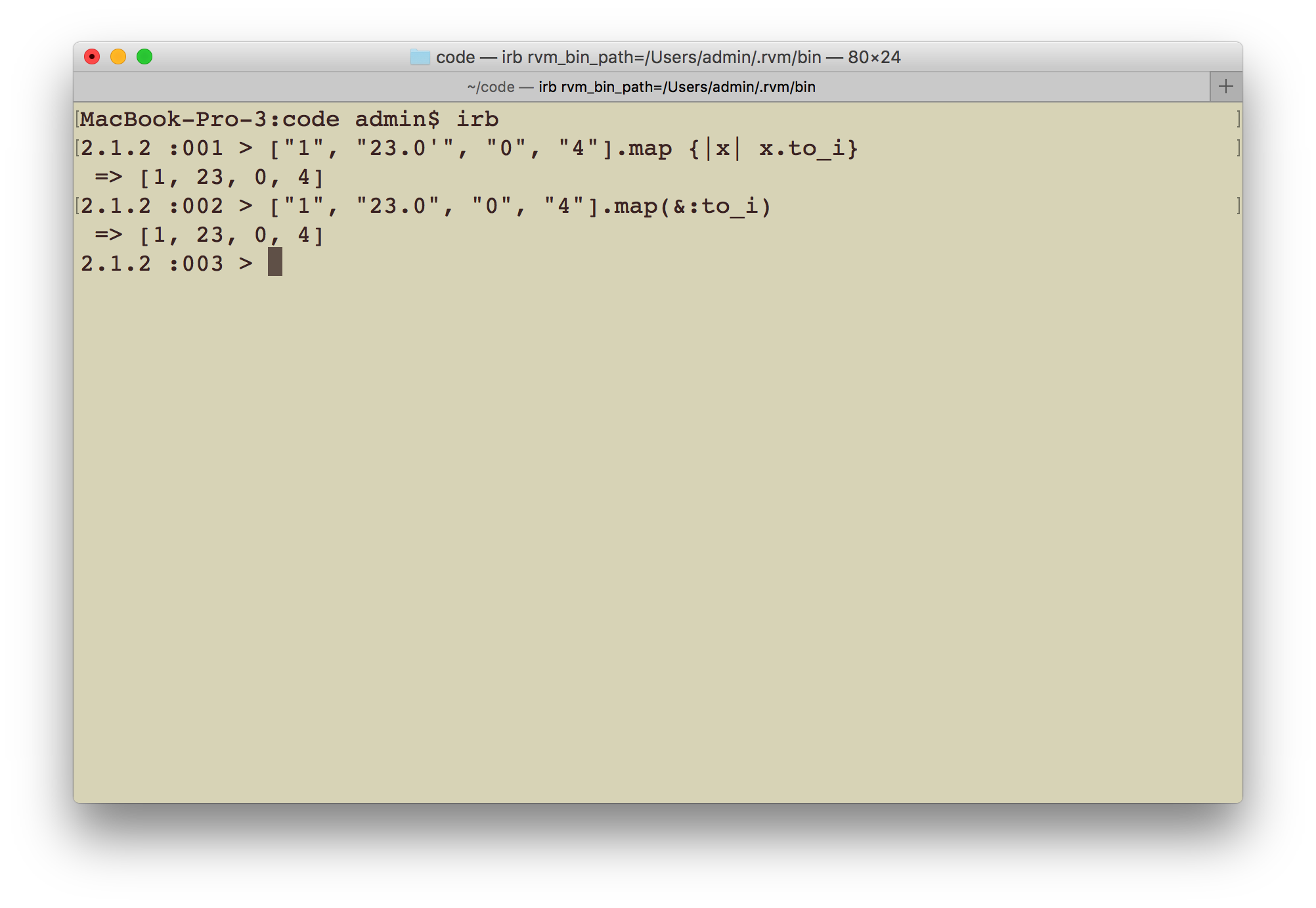How to Use the Map Method in Ruby - Part 1
In the next few lessons, we are going to be covering the map method - a popular method used by Ruby developers almost on an everyday basis due to its flexibility and usefulness.
• Watch Guide Video
Video locked
This video is viewable to users with a Bottega Bootcamp license

In the next few lessons, we are going to cover the map method, which is a popular iteration method used by Ruby developers on a regular basis due to its flexibility and usefulness.

For this guide I'm going to use the `irb` environment to show you the different environments available for Ruby. If you want to use this environment too, simply type `irb` in your terminal. Alternately, you can also use `Repl.it` if you prefer.

This is how you can access `irb` (which stands for Interactive Ruby Shell).## `map` Method Code Example

We are going to learn how to use the `map` method with a practical example. Let's imagine that we have an array of strings that represent numbers. This is a common occurrence in Ruby when you work with APIs since they always returns values in a string format (even numbers). In cases like these you may want to convert the values into actual integer data types so that you can use them in your application. This is a great example of how you can leverage the `map` method. Let's enter this code into the `irb` session:

```["1", "23.0", "0", "4"].map {|x| x.to_i}
```

In this code we have an array of strings that represent numbers. To convert each value to its integer equivalent, we're using a block variable `x` and passing the `to_i` method that convert each element to an integer. The output will be:If you remember back to our lesson on the `select` method you will remember that Ruby allows us to use the ampersand shortcut in cases like this. To leverage this syntactic sugar we can use the following code:

```["1", "23.0", "0", "4"].map(&:to_i)
```

Running this and you'll see that we will get the same result.Another example of the `map` method in action would be to double the string value. Let's say you have a list of letters like `a, b, c, d...` and you want to double each string, resulting in: `aa, bb, cc, dd...`. This is similar to what you may have seen in an Excel spreadsheet for representing columns.

To accomplish this feature we can use the following code:

```("a"..."g").map { |i| i * 2 }
```

In this code, we are declaring a collection that comprises of the letters `a` to `g`.

devCamp Note: the above notation is enough for Ruby to understand that the collection should start from `a` and end at `g` and leverages the same range functionality we've used with integers.

Inside of the `map` block we're declaring a block variable of `i` and multiplying each element by two. When you multiply a letter by two in Ruby it simply doubles the letter. So, the output is:

```["aa", "bb", "cc", "dd", "ee", "ff"]
```

Let's take a look at one another example with the `map` method. This time we are going to convert an array of numbers into a hash that has the key as the number itself and the value as the integer value of the number. If that sounds complicated don't worry, I simply want to to illustrate the power of the method, we can worry about what it means later on when we dive into the `Hash` data structure.

This is what the code will look like:

```Hash[[1, 2.1, 3.33, 0.9].map {|x| [x, x.to_i]}]
```

In this code, I'm creating a `Hash` data structure first. Next, I'm iterating through an array of numbers and mapping it to the hash with the `map` method. I'm using an iterator variable `x` to go through each array value. Next, I'm giving two values to the hash, where the first is the value itself, and second is the integer of that value. For example, if a number is `3.33`, then the key is `3.33` and value is the integer value of it, which is `3`.

This what the output looks like:

```{1=>1, 2.1=>2, 3.33=>3, 0.9=>0}
```

If you want to have the integer as the key and the actual number as the value, simply switch it up to look like this:

```Hash[[1, 2.1, 3.33, 0.9].map {|x| [x.to_i, x]}]
```

This will result in the following output:

```{1=>1, 2=>2.1, 3=>3.33, 0=>0.9}
```

So in this guide we've walked through three different ways to use the `map` method. In the next lesson we'll discuss how more advanced components that `map` provides.